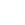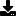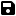4 different types of sorts (Bubble,Comb,Selection,Exchange)

by Peepy
Category: String Manipulation
Compatability: Visual Basic 4.0 (32-bit)
Difficulty: Unknown Difficulty
Originally Published: Wed 6 January 1999
Date Added: Mon 8 February 2021
Rating:(1 Votes)

4 different types of sorts (Bubble,Comb,Selection,Exchange)

Rate 4 different types of sorts (Bubble,Comb,Selection,Exchange)

Bubble sort
''
Dim I, J  As Integer
Dim Swapped As Boolean
Dim Temp As String
I = Arrsize
Do
Swapped = False
For J = 0 To I - 1
lstbubble.AddItem Array2(1) & "," & Array2(2) & "," & Array2(3) & "," & Array2(4) & "," & Array2(5)

Bubcom = Bubcom + 1
If Array2(J) > Array2(J + 1) Then 'swap

Temp = Array2(J)
Array2(J) = Array2(J + 1)
Array2(J + 1) = Temp

Swapped = True
Bubbleswap = Bubbleswap + 1
'lstbubble.AddItem Array2(1) & "," & Array2(2) & "," & Array2(3) & "," & Array2(4) & "," & Array2(5)
End If

Next J

I = I - 1
Loop Until Not Swapped
'''''
Exchange sort
''''''''''''''''''''''''''''''''''''
Dim Front, Back, I As Integer
Dim Temp As String

For Front = 0 To Arrsize
For Back = Front To Arrsize
Excom = Excom + 1

If Array(Front) > Array(Back) Then
Temp = Array(Front)
Array(Front) = Array(Back)
Array(Back) = Temp
Exswap = Exswap + 1
End If
Next Back
Next Front
'''''''''
Comb sort
''''''''' Dim I, J, Temp As Integer
Const Shrink = 1.3
Dim Gap As Single
Dim Swapped As Boolean
Gap = Arrsize - 1

Do
Gap = Int(Gap / Shrink)
Swapped = True
Combcom = Combcom + 1
For J = 0 To Arrsize - Gap
If Array3(J) > Array3(J + Gap) Then
Temp = Array3(J)
Array3(J) = Array3(J + Gap)
Array3(J + Gap) = Temp
Swapped = False
Combswap = Combswap + 1
End If
Next J
Loop Until Not Swapped And Gap = 1

For I = 0 To Arrsize
Next I
'''''''''''
Selection sort
'''''''''
Dim Front, Back, I, Loc As Integer
Dim Temp As String

For Front = 0 To Arrsize - 1
Loc = Front

For Back = Front To Arrsize
Selcom = Selcom + 1
If Array4(Loc) > Array4(Back) Then
Loc = Back
End If
Next Back
Selswap = Selswap + 1
Temp = Array4(Loc)
Array4(Loc) = Array4(Front)
Array4(Front) = Temp

Next FrontDownload this snippetAdd to My Saved Code